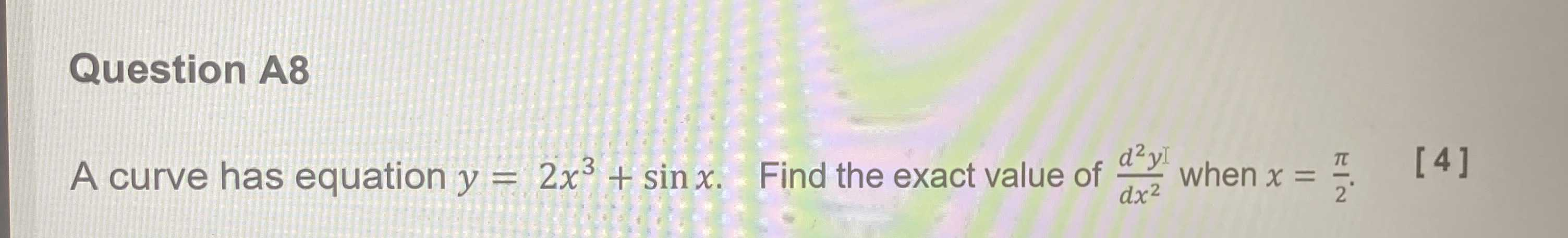### ¿Todavía tienes preguntas de matemáticas?

Pregunte a nuestros tutores expertos
Algebra
PreguntaA curve has equation $$y = 2 x ^ { 3 } + \sin x$$ . Find the exact value of $$\frac { d ^ { 2 } y } { d x ^ { 2 } }$$ when $$x = \frac { \pi } { 2 }$$ . 

$$= 6\pi- 1$$